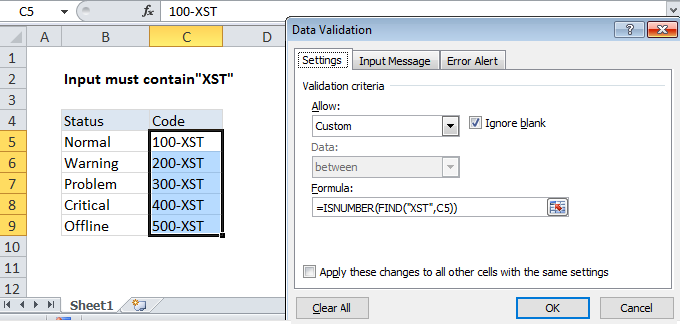## Excel Office

Excel How Tos, Tutorials, Tips & Tricks, Shortcuts

# Set criteria to reject specific data in Excel

To disallow input that contains one of many things, you can use a custom data validation rule based the SEARCH function.

## Formula

`=SUMPRODUCT(--ISNUMBER(SEARCH(list,A1)))=0`## Explanation

In the example shown above, the data validation applied to B5:B11 is:

`=SUMPRODUCT(--ISNUMBER(SEARCH(list,B5)))=0`

Note:  Cell references in data validation formulas are relative to the upper left cell in the range selected when the validation rule is defined, in this case B5.

Worked Example:   Sum if equal to either x or y in Excel

### How this formula works

Data validation rules are triggered when a user adds or changes a cell value.

This formula uses the SEARCH function to test user input for each value in the named range “list”. The search logic is “contains” — when a value from “list” is found is found, SEARCH returns the position of the value as a number. If not found, SEARCH returns an error.

Worked Example:   IF with wildcards in Excel

The ISNUMBER function then converts numbers to TRUE and errors to FALSE, and the double negative operator changes the TRUE FALSE values to 1s and zeros. Since the named range “list” contains 5 values, we get back 5 results in an array like this:

Worked Example:   Highlight numbers that include symbols in Excel

{0;0;0;0;0}

SUMPRODUCT then sums up the items in the array and the result is tested against zero. As long as all items are zero, SUMPRODUCT returns zero and validation succeeds. If SUMPRODUCT returns other number (i.e. when an item in “list” is found) the the formula returns FALSE and validation fails.